# ML Aggarwal Class 10 Solutions for ICSE Maths Chapter 5 Quadratic Equations in One Variable Ex 5.3

## ML Aggarwal Class 10 Solutions for ICSE Maths Chapter 5 Quadratic Equations in One Variable Ex 5.3

ML Aggarwal Class 10 Solutions for ICSE Maths Chapter 5 Quadratic Equations in One Variable Ex 5.3

Solve the following (1 to 8) equations by using the formula:

Question 1.
(i) 2x² – 7x + 6 = 0
(ii) 2x² – 6x + 3 = 0
Solution: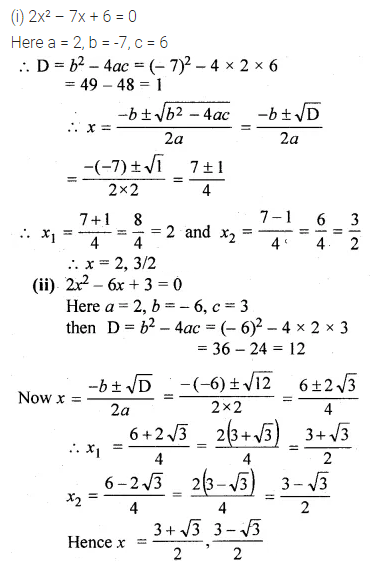Question 2.
(i) x² + 7x – 7 = 0
(ii) (2x + 3)(3x – 2) + 2 = 0
Solution: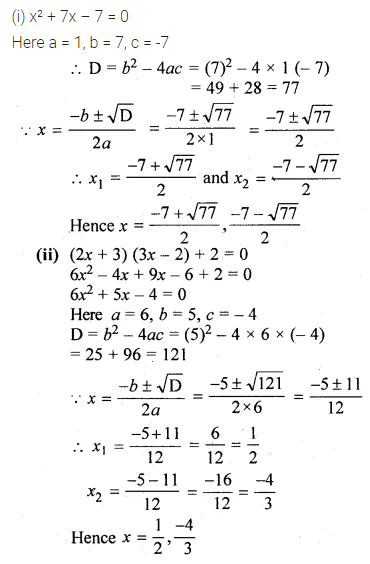Question 3.
(i) 256x² – 32x + 1 = 0
(ii) 25x² + 30x + 7 = 0
Solution:Question 4.
(i) 2x² + √5x – 5 = 0
(ii) √3x² + 10x – 8√3 = 0
Solution: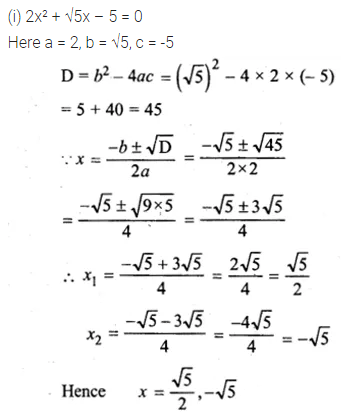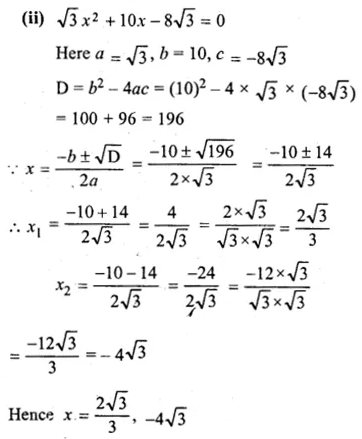Question 5.
(i) $$\frac { x-2 }{ x+2 } +\frac { x+2 }{ x-2 } =4$$
(ii) $$\frac { x+1 }{ x+3 } =\frac { 3x+2 }{ 2x+3 }$$
Solution: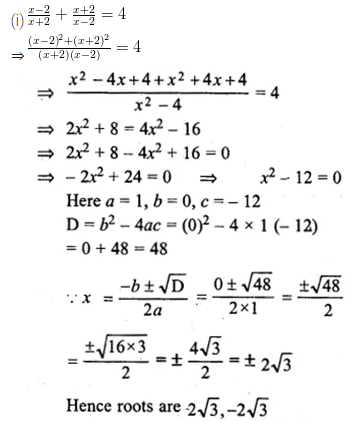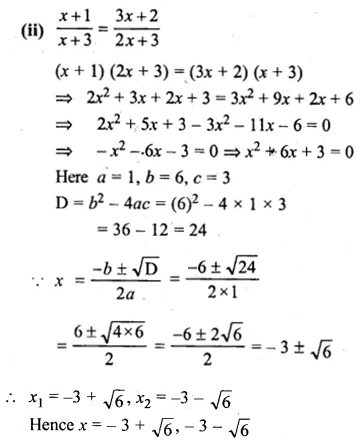Question 6.
(i) a (x² + 1) = (a² + 1) x , a ≠ 0
(ii) 4x² – 4ax + (a² – b²) = 0
Solution: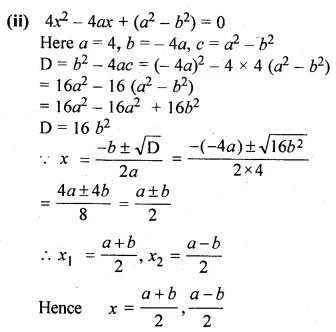Question 7.
(i)$$x-\frac { 1 }{ x } =3,x\neq 0$$
(ii)$$\frac { 1 }{ x } +\frac { 1 }{ x-2 } =3,x\neq 0,2$$
Solution: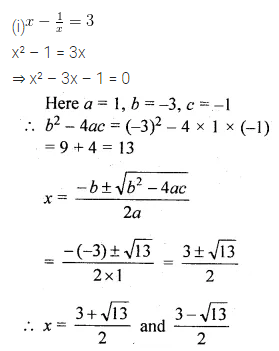Question 8.
$$\frac { 1 }{ x-2 } +\frac { 1 }{ x-3 } +\frac { 1 }{ x-4 } =0$$
Solution: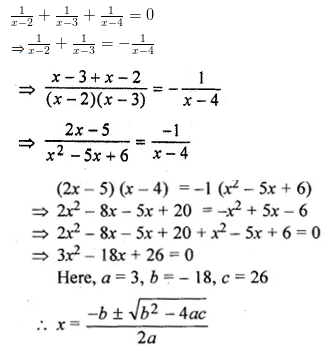Question 9.
Solve for $$x:2\left( \frac { 2x-1 }{ x+3 } \right) -3\left( \frac { x+3 }{ 2x-1 } \right) =5,x\neq -3,\frac { 1 }{ 2 }$$
Solution: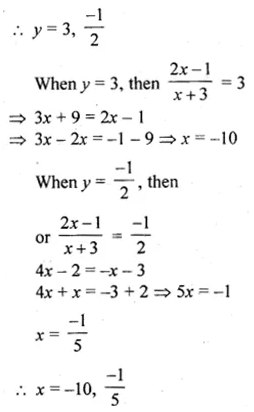Question 10.
Solve the following equation by using quadratic equations for x and give your
(i) x² – 5x – 10 = 0
(ii) 5x(x + 2) = 3
Solution:Question 11.
Solve the following equations by using quadratic formula and give your answer correct to 2 decimal places :
(i) 4x² – 5x – 3 = 0
(ii) 2x – $$\\ \frac { 1 }{ x }$$ = 1
Solution: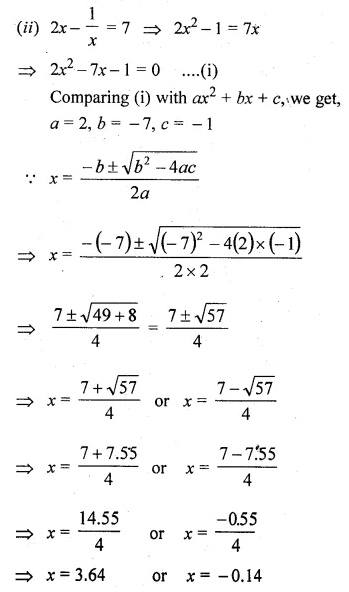Question 12.
Solve the following equation: $$x-\frac { 18 }{ x } =6$$. Give your answer correct to two x significant figures. (2011)
Solution: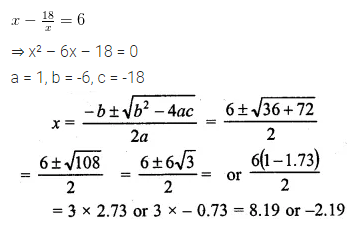Question 13.
Solve the equation 5x² – 3x – 4 = 0 and give your answer correct to 3 significant figures:
Solution: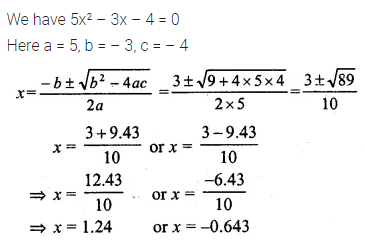ML Aggarwal Class 10 Solutions for ICSE Maths